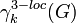# LCS-Lazard Lie group

BEWARE! This term is nonstandard and is being used locally within the wiki. [SHOW MORE]
This article defines a group property: a property that can be evaluated to true/false for any given group, invariant under isomorphism
View a complete list of group properties
VIEW RELATED: Group property implications | Group property non-implications |Group metaproperty satisfactions | Group metaproperty dissatisfactions | Group property satisfactions | Group property dissatisfactions

## Definition

A LCS-Lazard Lie group is a group$G$ satisfying both the following properties:

1. It is a 3-locally nilpotent group, i.e., any three elements of the group generate a nilpotent subgroup.
2. Its 3-local lower central series powering threshold is$\infty$. Explicitly, for any nonnegative integer$k$, let$\gamma_k^{3-loc}(G)$ denote the$k^{th}$ member of the 3-local lower central series of$G$. Then,$\gamma_k^{3-loc}(G)$ is powered over all the primes$p \le k$.

The definition of LCS-Lazard Lie group is somewhat nicer than the definition of Lazard Lie group in that it does not involve a "nilpotency class-specific definition."

## Metaproperties

Metaproperty name Satisfied? Proof Statement with symbols
subgroup-closed group property No LCS-Lazard Lie property is not subgroup-closed It is possible to have a LCS-Lazard Lie group$G$ and a subgroup$H$ of$G$ such that$H$ is not a Lazard Lie group.
quotient-closed group property No LCS-Lazard Lie property is not quotient-closed It is possible to have a LCS-Lazard Lie group$G$ and a normal subgroup$H$ of$G$ such that the quotient group$G/H$ is not a LCS-Lazard Lie group.
finite direct product-closed group property Yes LCS-Lazard Lie property is finite direct product-closed If$G_1$ and$G_2$ are LCS-Lazard Lie groups, then the external direct product$G_1 \times G_2$ is a LCS-Lazard Lie group.

## Relation with other properties

### Stronger properties

Property Meaning Proof of implication Proof of strictness (reverse implication failure) Intermediate notions
abelian group
Baer Lie group
LCS-Baer Lie group